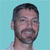## Data binding to an index property of a collection using WPFTags:
Databinding
IDataErrorInfo
LINQ
Windows Presentation Foundation
wpf
Hello: We are trying to do data binding to an element of collection thru an indexing property. However, the code does not seems to work. Please help. The following is the sample code: [The following is the code for the object in questions:] using System; using System.Collections.Generic; using System.Linq; using System.Text; using System.ComponentModel; using System.Xml.Linq; using System.Windows; namespace UIWpf { public class Person : IDataErrorInfo { private Guid id; public Guid Id { get{ return id; } set{ id = value; } } private int age; public int Age { get{ return age; } set{ age = value; } } private XElement data; public object this[string key] { get { return (from el in data.Elements() where el.Name == key select el.Value).First(); } set { data.Element(key).Value = value.ToString(); string a = ((IDataErrorInfo)this)["["+key+">">; } } public Person() { id = Guid.NewGuid(); age = 156; data = new XElement("Data", new XElement("Name", "Henstar"), new XElement("Description", "Hello, Henstar") ); } public void Save() { MessageBox.Show(data.ToString()); } string IDataErrorInfo.Error { get { return null; } } string IDataErrorInfo.this[string key] { get { string result = null; if (key == "Age") { if (this.age < 0 || this.age > 150) { result = "Age must not be less than 0 or greater than 150."; } } if (key == "[Name]") { if (data.Element("Name").Value.Length > 5) { result = "Name length can't exceed to 10"; } } return result; } } } } [The following is the code for the window class] <Window x:Class="UIWpf.Window1" xmlns="http://schemas.microsoft.com/winfx/2006/xaml/presentation" xmlns:x="http://schemas.microsoft.com/winfx/2006/xaml" Title="Window1" Height="300" Width="500"> <Grid> <Grid.Resources> </Grid.Resources> <StackPanel Name="splData"> <DockPanel Margin="10,10,10,10"> <Label Width="80">ID:</Label> <TextBox Width="200" Text="{Binding Path=Id, Mode=TwoWay, UpdateSourceTrigger=PropertyChanged, ValidatesOnDataErrors=True}" /> </DockPanel> <DockPanel Margin="10,10,10,10"> <Label Width="80">Age:</Label> <TextBox Width="200" Text="{Binding Path=Age, Mode=TwoWay, UpdateSourceTrigger=PropertyChanged, ValidatesOnDataErrors=True}" /> </DockPanel> <DockPanel Margin="10,10,10,10"> <Label Width="80">Name:</Label> <TextBox Width="200" Text="{Binding Path=[Name], Mode=TwoWay, UpdateSourceTrigger=PropertyChanged, ValidatesOnDataErrors=True}" /> </DockPanel> <DockPanel Margin="10,10,10,10"> <Label Width="80">Description:</Label> <TextBox Width="200" Text="{Binding Path=[Description], Mode=TwoWay, UpdateSourceTrigger=PropertyChanged, ValidatesOnDataErrors=True}" /> </DockPanel> <DockPanel Margin="10,10,10,10"> <Button Width="80" Click="Button_Click">Save</Button> </DockPanel> </StackPanel> </Grid> </Window> The symtom: The data binding work properly for Age but does not work for index property for [Name]. Please advice whether it is possible to do data binding to an element of a collection in the first place and if so, how can I get it to work properly. I am new to this so please forgive me if the question is a bid stupid. Thank.

Thanks. We'll let you know when a new response is added.
•